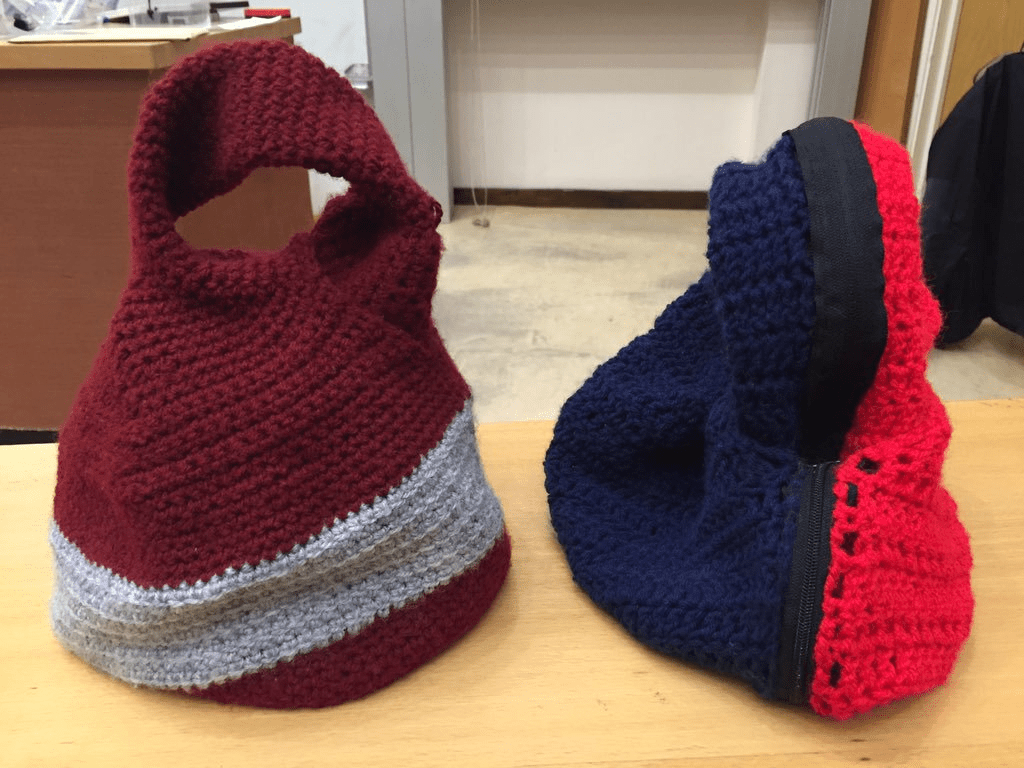# The wonders of mathematical crochet

Several months ago, I learned to crochet, and I’ve been hooked ever since. And as a mathematician, one of the most fantastic things about it is that it allows you to make mathematical objects that are enormously difficult to make, or even visualise in any other way.

For those of you unfamiliar with crochet, all you need is a ball of wool and a crochet hook. You then use this hook to pull loops of wool through other loops of wool, and from this you very quickly make a stretchy, slightly holey fabric. You can either use rows of stitches to make a rectangle, or you can begin in the middle of your work and make rounds of stitches that meet at either end.How to make a single crochet stitch

If you’re crocheting with rounds of stitches, the most obvious shape that you can make is a circle. But how do we construct a perfect circle?

Each single crochet stitch can be thought of as a square, albeit one that can be easily squashed or stretched. (I’m using US terminology here.) For the first round of stitches, we want to make a circle with radius $1$. Therefore, the circumference will be $2\pi$. In this case, we’ll take $\pi \approx 3$ for simplicity, and so in the first round we’ll have $6$ stitches.

When we add the second round, the radius of the circle is now $2$, and so we need $4\pi$ stitches in this round, which, using our crude approximation of $\pi$, is $12$ stitches. For the next round, the circle has radius $3$ and so the round has $18$ stitches. So in each round we add $2\pi$, or $6$, more stitches—the number of stitches in each round increases linearly.

If you try this and make a mistake in the number of stitches in a round, then you might notice that the circle stops being flat and starts to curve. This fact is actually what makes crochet such a powerful tool for creating mathematical objects—by changing the number of stitches then we change the curvature of the surface. The circle is flat, and therefore has zero curvature.

So what happens if we increase the number of stitches in a slower fashion? Each new round won’t stretch around the previous round, and hence we will create a surface with positive curvature. The classic example of a surface with positive curvature is a sphere—at every point on the sphere the surface curves away in all directions. To crochet a sphere, the number of stitches in each round increases like $\sin \theta$, where $\theta$ is the angle around the sphere. You can generate patterns for mathematically ideal crochet spheres here.

On the other hand, what if we increase the number of stitches in each round faster than linearly? For example, what if we double the number of stitches in each round so that the length of the rounds increases exponentially? Each round is much larger than the one before it, and so the surface has to curve up and down. By continuing this pattern, you create a hyperbolic pseudosphere, which a surface of constant negative curvature. This means that if you look at any point on the surface, it will look like a Pringle—curving upwards in one direction and downwards in the other.

Prior to 1997, it was extremely difficult to build a robust model of a hyperbolic pseudosphere, but then mathematician and crocheter Daina Taimina realised that you could easily construct one using crochet. The idea was hugely popular and led to a book, as well as a huge project creating coral reefs using hyperbolic crochet.

The following graph shows how the number of stitches in each round should increase when making a circle, sphere or hyperbolic surface.The existence of hyperbolic crochet is perhaps the best known example of mathematical crochet, but it only touches the surface of what crochet can represent. For example, an easy crochet project for beginners is a rectangular scarf. If you make a twist in the scarf and sew the ends together, you’ve made yourself a Möbius strip, which is a surface with only one side. (Try making one with some paper and drawing a line along the centre.) Apparently Alan Turing liked to knit Möbius strip scarves.Two crochet mobius strips with twists going in opposite directions. Zip them together to get a Klein bottle!

A particularly interesting fact about Möbius strips is that if you glue the edges of two strips together, then you create a Klein bottle. However, you can’t demonstrate this physically with paper, because it’s not stretchy or flexible enough. However this is actually a very easy project using crochet—I made one recently in an afternoon or two. An alternative way to think of a Klein bottle is to glue the ends of a tube together in opposite directions. I used this approach to crochet a Klein bottle hat, using the crochet lathe to generate a pattern. This clever tool gives you a pattern for any surface of revolution.Two crocheted klein bottles. The right hand one is made by zipping together two Mobius strips

Another classic crochet project is granny squares. With lots of these squares, you can then construct polyominoes, and make a mathematical/tetris blanket like this one.

A particularly impressive mathematical crochet feat is this model of the Lorenz manifold, created by researchers at the University of Auckland. The complexity and curvature of this surface means it is very difficult to create physically, but once again the flexibility of crochet came to the rescue.

And one final example of the possibilities of combining mathematics and crochet—hexaflexagons. Woolly Thoughts, who create beautiful mathematical knitting, have written a pattern for a crocheted hexaflexagon cushion.I hope I’ve convinced you to pick up a hook and get crocheting! While some of these patterns are very complex, a hyperbolic pseudosphere is very simple, and was actually the first thing I crocheted. (Many thanks to Matthew Scroggs for teaching me.) What other mathematical objects can you create with crochet?

Cover image by Cheryl on Flickr.